最短路径算法_最短路径算法比较 - CSDNShortest Path Algorithm

Dijkstra算法、Floyd算法等

• ## 最短路径问题---Dijkstra算法详解

万次阅读 多人点赞 2018-03-10 09:58:58
前言 Nobody can go back and start a new beginning,but anyone can start today and make a new ...从图中的某个顶点出发到达另外一个顶点的所经过的边的权重和最小的一条路径，称为最短路径 解决问题的算法：...
前言
Nobody can go back and start a new beginning,but anyone can start today and make a new ending.
Name:Willam
Time:2017/3/8

1、最短路径问题介绍

问题解释：
从图中的某个顶点出发到达另外一个顶点的所经过的边的权重和最小的一条路径，称为最短路径

解决问题的算法：

迪杰斯特拉算法（Dijkstra算法）
弗洛伊德算法（Floyd算法）
SPFA算法

这篇博客，我们就对Dijkstra算法来做一个详细的介绍

2、Dijkstra算法介绍

算法特点：

迪科斯彻算法使用了广度优先搜索解决赋权有向图或者无向图的单源最短路径问题，算法最终得到一个最短路径树。该算法常用于路由算法或者作为其他图算法的一个子模块。
算法的思路

Dijkstra算法采用的是一种贪心的策略，声明一个数组dis来保存源点到各个顶点的最短距离和一个保存已经找到了最短路径的顶点的集合：T，初始时，原点 s 的路径权重被赋为 0 （dis[s] = 0）。若对于顶点 s 存在能直接到达的边（s,m），则把dis[m]设为w（s, m）,同时把所有其他（s不能直接到达的）顶点的路径长度设为无穷大。初始时，集合T只有顶点s。
然后，从dis数组选择最小值，则该值就是源点s到该值对应的顶点的最短路径，并且把该点加入到T中，OK，此时完成一个顶点，
然后，我们需要看看新加入的顶点是否可以到达其他顶点并且看看通过该顶点到达其他点的路径长度是否比源点直接到达短，如果是，那么就替换这些顶点在dis中的值。
然后，又从dis中找出最小值，重复上述动作，直到T中包含了图的所有顶点。

3、Dijkstra算法示例演示

下面我求下图，从顶点v1到其他各个顶点的最短路径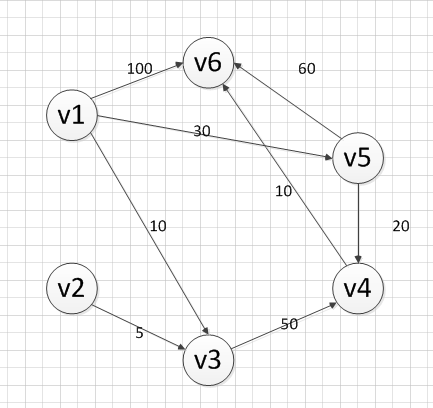首先第一步，我们先声明一个dis数组，该数组初始化的值为：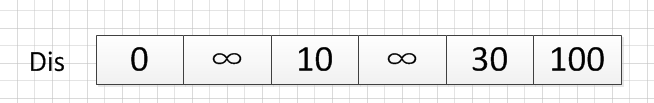我们的顶点集T的初始化为：T={v1}

既然是求 v1顶点到其余各个顶点的最短路程，那就先找一个离 1 号顶点最近的顶点。通过数组 dis 可知当前离v1顶点最近是 v3顶点。当选择了 2 号顶点后，dis（下标从0开始）的值就已经从“估计值”变为了“确定值”，即 v1顶点到 v3顶点的最短路程就是当前 dis值。将V3加入到T中。
为什么呢？因为目前离 v1顶点最近的是 v3顶点，并且这个图所有的边都是正数，那么肯定不可能通过第三个顶点中转，使得 v1顶点到 v3顶点的路程进一步缩短了。因为 v1顶点到其它顶点的路程肯定没有 v1到 v3顶点短.

OK，既然确定了一个顶点的最短路径，下面我们就要根据这个新入的顶点V3会有出度，发现以v3 为弧尾的有： < v3,v4 >,那么我们看看路径：v1–v3–v4的长度是否比v1–v4短，其实这个已经是很明显的了，因为dis代表的就是v1–v4的长度为无穷大，而v1–v3–v4的长度为：10+50=60，所以更新dis的值,得到如下结果：因此 dis要更新为 60。这个过程有个专业术语叫做“松弛”。即 v1顶点到 v4顶点的路程即 dis，通过 < v3,v4> 这条边松弛成功。这便是 Dijkstra 算法的主要思想：通过“边”来松弛v1顶点到其余各个顶点的路程。

然后，我们又从除dis和dis外的其他值中寻找最小值，发现dis的值最小，通过之前是解释的原理，可以知道v1到v5的最短距离就是dis的值，然后，我们把v5加入到集合T中，然后，考虑v5的出度是否会影响我们的数组dis的值，v5有两条出度：< v5,v4>和 < v5,v6>,然后我们发现：v1–v5–v4的长度为：50，而dis的值为60，所以我们要更新dis的值.另外，v1-v5-v6的长度为：90，而dis为100，所以我们需要更新dis的值。更新后的dis数组如下图：然后，继续从dis中选择未确定的顶点的值中选择一个最小的值，发现dis的值是最小的，所以把v4加入到集合T中，此时集合T={v1,v3,v5,v4},然后，考虑v4的出度是否会影响我们的数组dis的值，v4有一条出度：< v4,v6>,然后我们发现：v1–v5–v4–v6的长度为：60，而dis的值为90，所以我们要更新dis的值，更新后的dis数组如下图：然后，我们使用同样原理，分别确定了v6和v2的最短路径，最后dis的数组的值如下：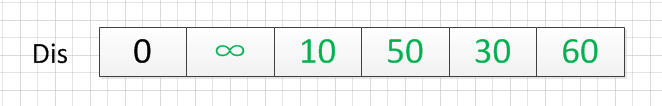因此，从图中，我们可以发现v1-v2的值为：∞，代表没有路径从v1到达v2。所以我们得到的最后的结果为：

起点  终点    最短路径    长度
v1    v2     无          ∞
v3     {v1,v3}    10
v4     {v1,v5,v4}  50
v5     {v1,v5}    30
v6     {v1，v5,v4,v6} 60


4、Dijkstra算法的代码实现（c++）

Dijkstra.h文件的代码

/************************************************************/
/*                程序作者：Willam                          */
/*                程序完成时间：2017/3/8                    */
/*                有任何问题请联系：2930526477@qq.com       */
/************************************************************/
//@尽量写出完美的程序

#pragma once
//#pragma once是一个比较常用的C/C++杂注，
//只要在头文件的最开始加入这条杂注，
//就能够保证头文件只被编译一次。

#include<iostream>
#include<string>
using namespace std;

/*
本程序是使用Dijkstra算法实现求解最短路径的问题
采用的邻接矩阵来存储图
*/
//记录起点到每个顶点的最短路径的信息
struct Dis {
string path;
int value;
bool visit;
Dis() {
visit = false;
value = 0;
path = "";
}
};

class Graph_DG {
private:
int vexnum;   //图的顶点个数
int edge;     //图的边数
int **arc;   //邻接矩阵
Dis * dis;   //记录各个顶点最短路径的信息
public:
//构造函数
Graph_DG(int vexnum, int edge);
//析构函数
~Graph_DG();
// 判断我们每次输入的的边的信息是否合法
//顶点从1开始编号
bool check_edge_value(int start, int end, int weight);
//创建图
void createGraph();
//打印邻接矩阵
void print();
//求最短路径
void Dijkstra(int begin);
//打印最短路径
void print_path(int);
};


Dijkstra.cpp文件的代码

#include"Dijkstra.h"

//构造函数
Graph_DG::Graph_DG(int vexnum, int edge) {
//初始化顶点数和边数
this->vexnum = vexnum;
this->edge = edge;
//为邻接矩阵开辟空间和赋初值
arc = new int*[this->vexnum];
dis = new Dis[this->vexnum];
for (int i = 0; i < this->vexnum; i++) {
arc[i] = new int[this->vexnum];
for (int k = 0; k < this->vexnum; k++) {
//邻接矩阵初始化为无穷大
arc[i][k] = INT_MAX;
}
}
}
//析构函数
Graph_DG::~Graph_DG() {
delete[] dis;
for (int i = 0; i < this->vexnum; i++) {
delete this->arc[i];
}
delete arc;
}

// 判断我们每次输入的的边的信息是否合法
//顶点从1开始编号
bool Graph_DG::check_edge_value(int start, int end, int weight) {
if (start<1 || end<1 || start>vexnum || end>vexnum || weight < 0) {
return false;
}
return true;
}

void Graph_DG::createGraph() {
cout << "请输入每条边的起点和终点（顶点编号从1开始）以及其权重" << endl;
int start;
int end;
int weight;
int count = 0;
while (count != this->edge) {
cin >> start >> end >> weight;
//首先判断边的信息是否合法
while (!this->check_edge_value(start, end, weight)) {
cout << "输入的边的信息不合法，请重新输入" << endl;
cin >> start >> end >> weight;
}
//对邻接矩阵对应上的点赋值
arc[start - 1][end - 1] = weight;
//无向图添加上这行代码
//arc[end - 1][start - 1] = weight;
++count;
}
}

void Graph_DG::print() {
cout << "图的邻接矩阵为：" << endl;
int count_row = 0; //打印行的标签
int count_col = 0; //打印列的标签
//开始打印
while (count_row != this->vexnum) {
count_col = 0;
while (count_col != this->vexnum) {
if (arc[count_row][count_col] == INT_MAX)
cout << "∞" << " ";
else
cout << arc[count_row][count_col] << " ";
++count_col;
}
cout << endl;
++count_row;
}
}
void Graph_DG::Dijkstra(int begin){
//首先初始化我们的dis数组
int i;
for (i = 0; i < this->vexnum; i++) {
//设置当前的路径
dis[i].path = "v" + to_string(begin) + "-->v" + to_string(i + 1);
dis[i].value = arc[begin - 1][i];
}
//设置起点的到起点的路径为0
dis[begin - 1].value = 0;
dis[begin - 1].visit = true;

int count = 1;
//计算剩余的顶点的最短路径（剩余this->vexnum-1个顶点）
while (count != this->vexnum) {
//temp用于保存当前dis数组中最小的那个下标
//min记录的当前的最小值
int temp=0;
int min = INT_MAX;
for (i = 0; i < this->vexnum; i++) {
if (!dis[i].visit && dis[i].value<min) {
min = dis[i].value;
temp = i;
}
}
//cout << temp + 1 << "  "<<min << endl;
//把temp对应的顶点加入到已经找到的最短路径的集合中
dis[temp].visit = true;
++count;
for (i = 0; i < this->vexnum; i++) {
//注意这里的条件arc[temp][i]!=INT_MAX必须加，不然会出现溢出，从而造成程序异常
if (!dis[i].visit && arc[temp][i]!=INT_MAX && (dis[temp].value + arc[temp][i]) < dis[i].value) {
//如果新得到的边可以影响其他为访问的顶点，那就就更新它的最短路径和长度
dis[i].value = dis[temp].value + arc[temp][i];
dis[i].path = dis[temp].path + "-->v" + to_string(i + 1);
}
}
}

}
void Graph_DG::print_path(int begin) {
string str;
str = "v" + to_string(begin);
cout << "以"<<str<<"为起点的图的最短路径为：" << endl;
for (int i = 0; i != this->vexnum; i++) {
if(dis[i].value!=INT_MAX)
cout << dis[i].path << "=" << dis[i].value << endl;
else {
cout << dis[i].path << "是无最短路径的" << endl;
}
}
}

main.cpp文件的代码

#include"Dijkstra.h"

//检验输入边数和顶点数的值是否有效，可以自己推算为啥：
//顶点数和边数的关系是：((Vexnum*(Vexnum - 1)) / 2) < edge
bool check(int Vexnum, int edge) {
if (Vexnum <= 0 || edge <= 0 || ((Vexnum*(Vexnum - 1)) / 2) < edge)
return false;
return true;
}
int main() {
int vexnum; int edge;

cout << "输入图的顶点个数和边的条数：" << endl;
cin >> vexnum >> edge;
while (!check(vexnum, edge)) {
cout << "输入的数值不合法，请重新输入" << endl;
cin >> vexnum >> edge;
}
Graph_DG graph(vexnum, edge);
graph.createGraph();
graph.print();
graph.Dijkstra(1);
graph.print_path(1);
system("pause");
return 0;
}

输入：

6 8
1 3 10
1 5 30
1 6 100
2 3 5
3 4 50
4 6 10
5 6 60
5 4 20

输出：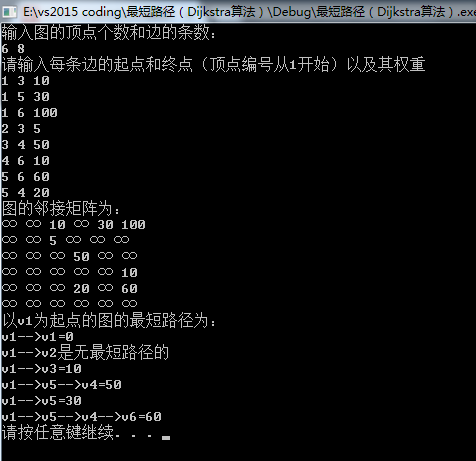从输出可以看出，程序的结果和我们之前手动计算的结果是一样的。
展开全文算法 数据结构 最短路径问题 Dijkstra算法
• 本文总结了图的几种最短路径算法的实现：深度或广度优先搜索算法，弗洛伊德算法，迪杰斯特拉算法，Bellman-Ford算法1），深度或广度优先搜索算法（解决单源最短路径）从起始结点开始访问所有的深度遍历路径或广度...
本文总结了图的几种最短路径算法的实现：深度或广度优先搜索算法，弗洛伊德算法，迪杰斯特拉算法，Bellman-Ford算法1），深度或广度优先搜索算法（解决单源最短路径）从起始结点开始访问所有的深度遍历路径或广度优先路径，则到达终点结点的路径有多条，取其中路径权值最短的一条则为最短路径。下面是核心代码：void dfs(int cur, int dst){
/***operation***/

/***operation***/
if(minPath < dst) return;//当前走过路径大于之前最短路径，没必要再走下去
if(cur == n){//临界条件
if(minPath > dst) minPath = dst;
return;
}
else{
int i;
for(i = 1; i <= n; i++){
if(edge[cur][i] != inf && edge[cur][i] != 0 && mark[i] == 0){
mark[i] = 1;
dfs(i, dst+edge[cur][i]);
mark[i] = 0;  //需要在深度遍历返回时将访问标志置0
}
}
return;
}
}例1：下面是城市的地图，注意是单向图，求城市1到城市5的最短距离。(引用的是上次总结的图论（一）中1）的例2)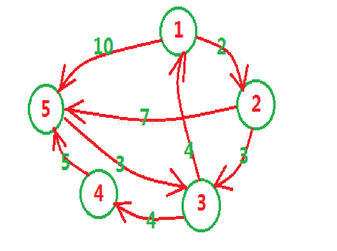/***先输入n个结点，m条边，之后输入有向图的m条边，边的前两元素表示起始结点，第三个值表权值，输出1号城市到n号城市的最短距离***/
/***算法的思路是访问所有的深度遍历路径，需要在深度遍历返回时将访问标志置0***/
#include <iostream>
#include <iomanip>
#define nmax 110
#define inf 999999999
using namespace std;
int n, m, minPath, edge[nmax][nmax], mark[nmax];//结点数，边数，最小路径，邻接矩阵，结点访问标记
void dfs(int cur, int dst){
/***operation***/

/***operation***/
if(minPath < dst) return;//当前走过路径大于之前最短路径，没必要再走下去
if(cur == n){//临界条件
if(minPath > dst) minPath = dst;
return;
}
else{
int i;
for(i = 1; i <= n; i++){
if(edge[cur][i] != inf && edge[cur][i] != 0 && mark[i] == 0){
mark[i] = 1;
dfs(i, dst+edge[cur][i]);
mark[i] = 0;
}
}
return;
}
}

int main(){
while(cin >> n >> m && n != 0){
//初始化邻接矩阵
int i, j;
for(i = 1; i <= n; i++){
for(j = 1; j <= n; j++){
edge[i][j] = inf;
}
edge[i][i] = 0;
}
int a, b;
while(m--){
cin >> a >> b;
cin >> edge[a][b];
}
//以dnf(1)为起点开始递归遍历
memset(mark, 0, sizeof(mark));
minPath = inf;
mark = 1;
dfs(1, 0);
cout << minPath << endl;
}
return 0;
}程序运行结果如下：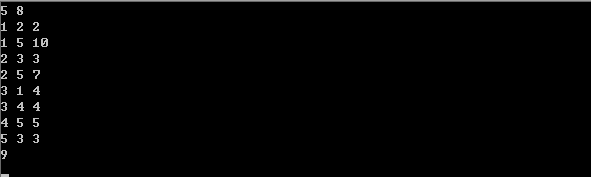2），弗洛伊德算法（解决多源最短路径）：时间复杂度O(n^3),空间复杂度O(n^2)基本思想：最开始只允许经过1号顶点进行中转，接下来只允许经过1号和2号顶点进行中转......允许经过1~n号所有顶点进行中转，来不断动态更新任意两点之间的最短路程。即求从i号顶点到j号顶点只经过前k号点的最短路程。分析如下：1，首先构建邻接矩阵Floyd[n+1][n+1]，假如现在只允许经过1号结点，求任意两点间的最短路程，很显然Floyd[i][j] = min{Floyd[i][j], Floyd[i]+Floyd[j]}，代码如下：for(i = 1; i <= n; i++){
for(j = 1; j <= n; j++){
if(Floyd[i][j] > Floyd[i] + Floyd[j])
Floyd[i][j] = Floyd[i] + Floyd[j];
}
}  2，接下来继续求在只允许经过1和2号两个顶点的情况下任意两点之间的最短距离，在已经实现了从i号顶点到j号顶点只经过前1号点的最短路程的前提下，现在再插入第2号结点，来看看能不能更新更短路径，故只需在步骤1求得的Floyd[n+1][n+1]基础上，进行Floyd[i][j] = min{Floyd[i][j], Floyd[i]+Floyd[j]}；......3，很显然，需要n次这样的更新，表示依次插入了1号，2号......n号结点，最后求得的Floyd[n+1][n+1]是从i号顶点到j号顶点只经过前n号点的最短路程。故核心代码如下：#define inf 99999999
for(k = 1; k <= n; k++){
for(i = 1; i <= n; i++){
for(j = 1; j <= n; j++){
if(Floyd[i][k] < inf && Floyd[k][j] < inf && Floyd[i][j] > Floyd[i][k] + Floyd[k][j])
Floyd[i][j] = Floyd[i][k] + Floyd[k][j];
}
}
}  例1：寻找最短的从商店到赛场的路线。其中商店在1号结点处，赛场在n号结点处，1~n结点中有m条线路双向连接。/***先输入n，m，再输入m个三元组，n为路口数，m表示有几条路其中1为商店，n为赛场，三元组分别表起点，终点，该路径长，输出1到n的最短路径***/
#include <iostream>
using namespace std;
#define inf 99999999
#define nmax 110
int edge[nmax][nmax], n, m;
int main(){
while(cin >> n >> m && n!= 0){
//构建邻接矩阵
int i, j;
for(i = 1; i <= n; i++){
for(j = 1; j <= n; j++){
edge[i][j] = inf;
}
edge[i][i] = 0;
}
while(m--){
cin >> i >> j;
cin >> edge[i][j];
edge[j][i] = edge[i][j];
}
//使用弗洛伊德算法
int k;
for(k = 1; k <= n; k++){
for(i = 1; i <= n; i++){
for(j = 1; j <= n; j++){
if(edge[i][k] < inf && edge[k][j] < inf && edge[i][j] > edge[i][k] + edge[k][j])
edge[i][j] = edge[i][k] + edge[k][j];
}
}
}
cout << edge[n] << endl;
}
return 0;
}程序运行结果如下：3），迪杰斯特拉算法（解决单源最短路径）基本思想：每次找到离源点（如1号结点）最近的一个顶点，然后以该顶点为中心进行扩展，最终得到源点到其余所有点的最短路径。基本步骤：1，设置标记数组book[]：将所有的顶点分为两部分,已知最短路径的顶点集合P和未知最短路径的顶点集合Q，很显然最开始集合P只有源点一个顶点。book[i]为1表示在集合P中；2，设置最短路径数组dst[]并不断更新：初始状态下，令dst[i] = edge[s][i](s为源点，edge为邻接矩阵)，很显然此时dst[s]=0，book[s]=1。此时，在集合Q中可选择一个离源点s最近的顶点u加入到P中。并依据以u为新的中心点，对每一条边进行松弛操作(松弛是指由结点s-->j的途中可以经过点u，并令dst[j]=min{dst[j], dst[u]+edge[u][j]})，并令book[u]=1;3，在集合Q中再次选择一个离源点s最近的顶点v加入到P中。并依据v为新的中心点，对每一条边进行松弛操作(即dst[j]=min{dst[j], dst[v]+edge[v][j]}),并令book[v]=1;4，重复3，直至集合Q为空。以下是图示：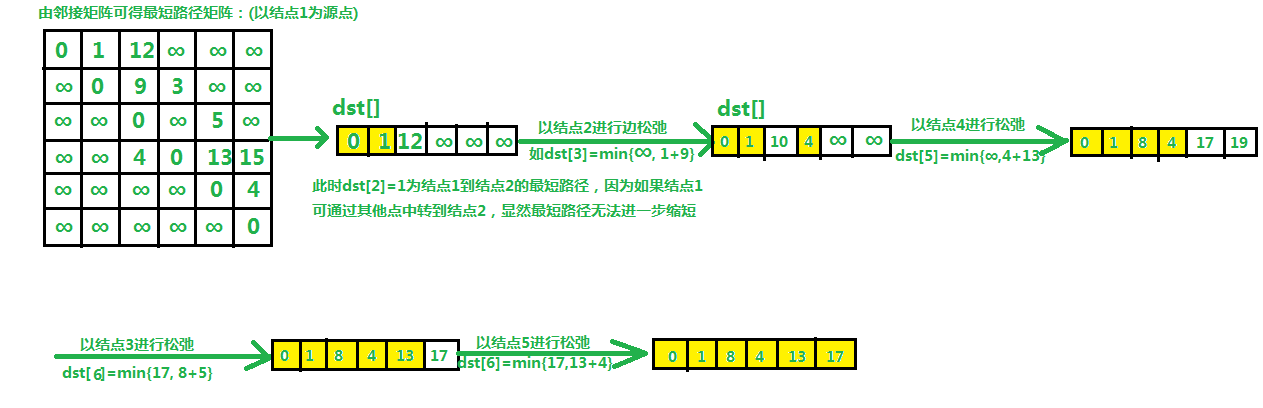核心代码如下所示：#define inf 99999999
/***构建邻接矩阵edge[][],且1为源点***/
for(i = 1; i <= n; i++) dst[i] = edge[s]；
for(i = 1; i <= n; i++) book[i] = 0;
book = 1;
for(i = 1; i <= n-1; i++){
//找到离源点最近的顶点u，称它为新中心点
min = inf;
for(j = 1; j <= n; j++){
if(book[j] == 0 && dst[j] < min){
min = dst[j];
u = j;
}
}
book[u] = 1;
//更新最短路径数组
for(k = 1; k <= n; k++){
if(edge[u][k] < inf && book[k] == 0){
if(dst[k] > dst[u] + edge[u][k])
dst[k] = dst[u] + edge[u][k];
}
}
}例1：给你n个点，m条无向边，每条边都有长度d和花费p，给你起点s，终点t，要求输出起点到终点的最短距离及其花费，如果最短距离有多条路线，则输出花费最少的。输入：输入n,m，点的编号是1~n,然后是m行，每行4个数 a,b,d,p，表示a和b之间有一条边，且其长度为d，花费为p。最后一行是两个数s,t;起点s，终点 t。n和m为 0 时输入结束。(1<n<=1000, 0<m<100000, s != t)输出：输出一行，有两个数， 最短距离及其花费。分析：由于每条边有长度d和花费p，最好构建边结构体存放，此外可以使用邻接链表，使用邻接链表时需要将上面的核心代码修改几个地方：1，初始化dst[]时使用结点1的邻接链表；2，更新最短路径数组时，k的范围由1~n变为1~edge[u].size()。先采用邻接矩阵解决此题，再使用邻接表解决此题，两种方法的思路都一样：初始化邻接矩阵或邻接链表，并初始化最短路径数组dst ----> n-1轮边的松弛中，先找到离新源点最近的中心点u，之后根据中心点u为转折点来更新路径数组。使用邻接矩阵求解：/***对于无向图，输入n,m，点的编号是1~n,然后是m行，每行4个数 a,b,d,p，表示a和b之间有一条边，且其长度为d，花费为p。最后一行是两个数s,t;起点s，终点 t。***/
/***n和m为 0 时输入结束。(1<n<=1000, 0<m<100000, s != t)     输出：输出一行，有两个数， 最短距离及其花费。***/
#include <iostream>
#include <iomanip>
using namespace std;
#define nmax 1001
#define inf 99999999
struct Edge{
int len;
int cost;
};
Edge edge[nmax][nmax];
int dst[nmax], spend[nmax], book[nmax], n, m, stNode, enNode;
int main(){
while(cin >> n >> m && n != 0 && m != 0){
int a, b, i, j;
//构建邻接矩阵和最短路径数组
for(i = 1; i <= n; i++){
for(j = 1; j <= n; j++){
edge[i][j].cost = 0;
edge[i][j].len = inf;
}
edge[i][i].len = 0;
}
while(m--){
cin >> a >> b;
cin >> edge[a][b].len >> edge[a][b].cost;
edge[b][a].len = edge[a][b].len;
edge[b][a].cost = edge[a][b].cost;
}
cin >> stNode >> enNode;
for(i = 1; i <= n; i++){
dst[i] = edge[stNode][i].len;
spend[i] = edge[stNode][i].cost;
}
memset(book, 0, sizeof(book));
book[stNode] = 1;
//开始迪杰斯特拉算法，进行剩余n-1次松弛
int k;
for(k = 1; k <= n-1; k++){
//找离源点最近的顶点u
int minNode, min = inf;
for(i = 1; i <= n; i++){
if(book[i] == 0 && min > dst[i] /* || min == dst[i]&& edge[stNode][min].cost > edge[stNode][i].cost*/){
min = dst[i];
minNode = i;
}
}
//cout << setw(2) << minNode;
book[minNode] = 1;//易错点1，错写成book[i]=1
//以中心点u为转折点来更新路径数组和花费数组
for(i = 1; i <= n; i++){
if(book[i] == 0 && dst[i] > dst[minNode] + edge[minNode][i].len || dst[i] == dst[minNode] + edge[minNode][i].len && spend[i] > spend[minNode] + edge[minNode][i].cost){
dst[i] = dst[minNode] + edge[minNode][i].len;//易错点2，错写成dst[i]+
spend[i] = spend[minNode] + edge[minNode][i].cost;
}
}
}
cout << dst[enNode] << setw(3) << spend[enNode] << endl;
}
return 0;
} 程序运行结果如下：使用邻接链表求解：/***对于无向图，输入n,m，点的编号是1~n,然后是m行，每行4个数 a,b,d,p，表示a和b之间有一条边，且其长度为d，花费为p。最后一行是两个数s,t;起点s，终点 t。***/
/***n和m为 0 时输入结束。(1<n<=1000, 0<m<100000, s != t)     输出：输出一行，有两个数， 最短距离及其花费。***/
#include <iostream>
#include <iomanip>
#include <vector>
using namespace std;
#define nmax 1001
#define inf 99999999
struct Edge{
int len;
int cost;
int next;
};
vector<Edge> edge[nmax];
int dst[nmax], spend[nmax], book[nmax], n, m, stNode, enNode;
int main(){
while(cin >> n >> m && n != 0 && m != 0){
int a, b, i, j;
//构建邻接表和最短路径数组
for(i = 1; i <= n; i++) edge[i].clear();
while(m--){
Edge tmp;
cin >> a >> b;
tmp.next = b;
cin >> tmp.len >> tmp.cost;
edge[a].push_back(tmp);
tmp.next = a;
edge[b].push_back(tmp);
}
cin >> stNode >> enNode;
for(i = 1; i <= n; i++) dst[i] = inf; //注意2，别忘记写此句来初始化dst[]
for(i = 0; i < edge[stNode].size(); i++){//注意1，从下标0开始存元素，误写成i <= edge[stNode].size()
dst[edge[stNode][i].next] = edge[stNode][i].len;
//cout << dst << endl;
spend[edge[stNode][i].next] = edge[stNode][i].cost;
}
memset(book, 0, sizeof(book));
book[stNode] = 1;
//开始迪杰斯特拉算法，进行剩余n-1次松弛
int k;
for(k = 1; k <= n-1; k++){
//找离源点最近的顶点u
int minnode, min = inf;
for(i = 1; i <= n; i++){
if(book[i] == 0 && min > dst[i] /* || min == dst[i]&& edge[stnode][min].cost > edge[stnode][i].cost*/){
min = dst[i];
minnode = i;
}
}
//cout << setw(2) << minnode;
book[minnode] = 1;//易错点1，错写成book[i]=1
//以中心点u为转折点来更新路径数组和花费数组
for(i = 0; i < edge[minnode].size(); i++){
int t = edge[minnode][i].next;//别忘了加此句，表示与结点minnode相邻的点
if(book[t] == 0 && dst[t] > dst[minnode] + edge[minnode][i].len || dst[t] == dst[minnode] + edge[minnode][i].len && spend[t] > spend[minnode] + edge[minnode][i].cost){
dst[t] = dst[minnode] + edge[minnode][i].len;
spend[t] = spend[minnode] + edge[minnode][i].cost;
}
}
}
cout << dst[enNode] << setw(3) << spend[enNode] << endl;
}
return 0;
}  程序运行结果如下：使用邻接表时，注意更新dst[]，book[]时要使用邻接表元素对应下标中的next成员，而涉及到权值加减时时需要使用邻接表中的对应下标来取得权值；而使用邻接矩阵就没这么多顾虑了，因为这时候邻接矩阵对应下标和dst[]要更新元素的下标正好一致，都是从1开始编号。4），Bellman-Ford算法(解决负权边，解决单源最短路径，前几种方法不能求含负权边的图)：：时间复杂度O(nm),空间复杂度O(m)主要思想：对所有的边进行n-1轮松弛操作，因为在一个含有n个顶点的图中，任意两点之间的最短路径最多包含n-1边。换句话说，第1轮在对所有的边进行松弛后，得到的是从1号顶点只能经过一条边到达其余各定点的最短路径长度。第2轮在对所有的边进行松弛后，得到的是从1号顶点只能经过两条边到达其余各定点的最短路径长度，......以下是图示：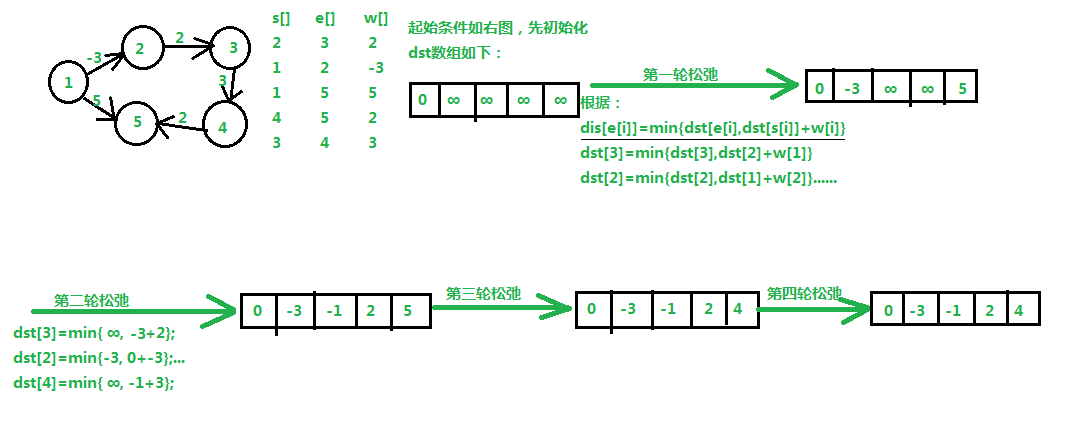此外，Bellman_Ford还可以检测一个图是否含有负权回路：如果在进行n-1轮松弛后仍然存在dst[e[i]] > dst[s[i]]+w[i]。算法核心代码如下：#define inf 999999999
for(i = 1; i <= n; i++) dst[i] = inf;
dst = 0;
for(k = 1; k <= n-1; k++){
for(i = 1; i <= m; i++){
if(dst[e[i]] > dst[s[i]] + w[i])
dst[e[i]] = dst[s[i]] + w[i];
}
}
//检测负权回路
flag = 0;
for(i = 1; i <= m; i++){
if(dst[e[i]] > dst[s[i]] + w[i])
flag = 1;
}
if(flag) cout << "此图含有负权回路";  例1：对图示中含负权的有向图，输出从结点1到各结点的最短路径，并判断有无负权回路。/***先输入n，m，分别表结点数和边数，之后输入m个三元组，各表起点，终点，边权，输出1号结点到各结点的最短路径****/
#include <iostream>
#include <iomanip>
using namespace std;
#define nmax 1001
#define inf 99999999
int n, m, s[nmax], e[nmax], w[nmax], dst[nmax];
int main(){
while(cin >> n >> m && n != 0 && m != 0){
int i, j;
//初始化三个数组：起点数组s[],终点数组e[],权值数组w[],最短路径数组dst[]
for(i = 1; i <= m; i++)
cin >> s[i] >> e[i] >> w[i];
for(i = 1; i <= n; i++)
dst[i] = inf;
dst = 0;
//使用Bellman_Ford算法
for(j = 1; j <= n-1; j++){
for(i = 1; i <= m; i++){
if(dst[e[i]] > dst[s[i]] + w[i])
dst[e[i]] = dst[s[i]] + w[i];
}
}
//测试是否有负权回路并输出
int flag = 0;
for(i = 1; i <= m; i++)
if(dst[e[i]] > dst[s[i]] + w[i])
flag = 1;
if(flag) cout << "此图含有负权回路\n";
else{
for(i = 1; i <= n; i++){
if(i == 1)
cout << dst[i];
else
cout << setw(3) << dst[i];
}
cout << endl;
}
}
return 0;
}  程序运行结果如下：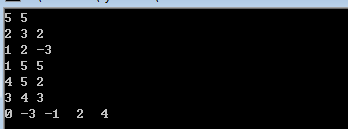展开全文• 1、单源点的最短路径问题：给定带权有向图G和源点v，求从v到G中其余各顶点的最短路径。 我们用一个例子来具体说明迪杰斯特拉算法的流程。 定义源点为 0，dist[i]为源点 0 到顶点 i 的最短路径。其过程描述如下...
一、Dijkstra算法
1、单源点的最短路径问题：给定带权有向图G和源点v，求从v到G中其余各顶点的最短路径。
我们用一个例子来具体说明迪杰斯特拉算法的流程。
定义源点为 0，dist[i]为源点 0 到顶点 i 的最短路径。其过程描述如下：

步骤
dist
dist
dist
dist
已找到的集合

第 1 步
8
1
2
+∞
{2}

第 2 步
8
×
2
4
{2, 3}

第 3 步
5
×
×
4
{2, 3, 4}

第 4 步
5
×
×
×
{2, 3, 4, 1}

第 5 步
×
×
×
×
{2, 3, 4, 1}

第 1 步：从源点 0 开始，找到与其邻接的点：1，2，3，更新dist[]数组，因 0 不与 4 邻接，故dist为正无穷。在dist[]中找到最小值，其顶点为 2，即此时已找到 0 到 2 的最短路。
第 2 步：从 2 开始，继续更新dist[]数组：2 与 1 不邻接，不更新；2 与 3 邻接，因0→2→3比dist大，故不更新dist ；2 与 4 邻接，因0→2→4比dist小，故更新dist为 4。在dist[]中找到最小值，其顶点为 3，即此时又找到 0 到 3 的最短路。
第 3 步：从 3 开始，继续更新dist[]数组：3 与 1 邻接，因0→3→1比dist小，更新dist为 5；3 与 4 邻接，因0→3→4比dist大，故不更新。在dist[]中找到最小值，其顶点为 4，即此时又找到 0 到 4 的最短路。
第 4 步：从 4 开始，继续更新dist[]数组：4 与 1 不邻接，不更新。在dist[]中找到最小值，其顶点为 1，即此时又找到 0 到 1 的最短路。
第 5 步：所有点都已找到，停止。
对于上述步骤，你可能存在以下的疑问：
若 A 作为源点，与其邻接的只有 B，C，D 三点，其dist[]最小时顶点为 C，即就可以确定A→C为 A 到 C 的最短路。但是我们存在疑问的是：是否还存在另一条路径使 A 到 C 的距离更小？ 用反证法证明。
假设存在如上图的红色虚线路径，使A→D→C的距离更小，那么A→D作为A→D→C的子路径，其距离也比A→C小，这与前面所述 “dist[]最小时顶点为 C” 矛盾，故假设不成立。因此这个疑问不存在。
根据上面的证明，我们可以推断出，Dijkstra 每次循环都可以确定一个顶点的最短路径，故程序需要循环 n-1 次。
/*
迪杰斯特拉求单节点到其余各节点的最短路径。
visited数组用于保存顶点是否已经求过最短路径，pre数组用于保存最短路径的下标
dist数组用于保存初始节点到其余节点的最短路径长度。
该算法求有向图G的某顶点到其余节点的最短路径pre以及长度dist
pre[v]的值是v0-->...->v的路径中的前驱节点。D[v]表示v0-->...-->v的最短路径长度和。

可以证明迪杰斯特拉算法每次循环可以确定一个顶点的最短路径，所以主程序循环n-1次。
主程序循环主要做两件事：首先找出dist数组中的最小值，并记录下标，说明找到初始点到该下标的最短路径。
然后要比价初始点到该点的最短路径加上这点到其他初始点需要到的点的距离是否比初始点直接到这些点的距离短
如果要短，那么就更新dist数组，并且这些点的前驱节点就会变为v而不是开始的v0点。下一次主循环再去从dist中找
最小的值并且未求过的点，就是该点的最短路径。
*/
#include<iostream>
using namespace std;
int matrix;//邻接矩阵
bool visited;//标记数组
int dist;//原点到i顶点的最短距离
int pre;//记录最短路径。pre[i]放的是i的前驱节点
int source;//源节点
int vertex_num;//顶点数
int edge_num;//边数

void Dijkstra(int source)
{
//首先初始化
memset(visited,0,sizeof(visited));
visited[source] = true;
for (int i = 0; i < vertex_num; i++)
{
dist[i] = matrix[source][i];
pre[i] = source;
}

int min_cost;//最短距离
int min_cost_index;//权值最小的那个顶点的下标。（求好了）
//主循环
for (int i = 1; i < vertex_num; i++)
{
min_cost = INT_MAX;
for (int j = 0; j < vertex_num; j++)
{
//注意要确保这个点没有找过。
if (visited[j]==false&&dist[j] < min_cost)
{
min_cost_index = j;
min_cost = dist[j];
}
}

visited[min_cost_index] = true;//找到某一个点的最短距离
//利用该点进行dist的更新，并且调整前驱。
for (int j = 0; j < vertex_num; j++)
{
//确保有连接
if (visited[j] == false && matrix[min_cost_index][j] != INT_MAX&&min_cost+ matrix[min_cost_index][j] < dist[j])
{
dist[j] = min_cost + matrix[min_cost_index][j];
pre[j] = min_cost_index;
}
}
}
}

int main()
{
cout << "请输入图的顶点数（<100）:";
cin >> vertex_num;
cout << "请输出图的边数: ";
cin >> edge_num;
for (int i = 0; i < vertex_num; i++)
{
for (int j = 0; j < vertex_num; j++)
{
matrix[i][j] = (i != j) ? INT_MAX : 0;
}
}
cout << "请输入边的信息：\n";
int u, v, w;
for (int i = 0; i < edge_num; i++)
{
cin >> u >> v >> w;
matrix[u][v] = matrix[v][u] = w;
}

cout << "请输入源点(<" << vertex_num << "): ";
cin >> source;
Dijkstra(source);
for (int i = 0; i < vertex_num; i++)
{
if (i != source)
{
//路径是反的，从目标点向前不断找前驱的过程。
cout << source << "到" << i << "最短距离： " << dist[i] << ",路径是：" << i;
int t = pre[i];
while (t != source)
{
cout << "--" << t;
t = pre[t];
}
cout << "--" << source << endl;
}
}
return 0;
}

二、Floyd算法暑假，小哼准备去一些城市旅游。有些城市之间有公路，有些城市之间则没有，如下图。为了节省经费以及方便计划旅程，小哼希望在出发之前知道任意两个城市之前的最短路程。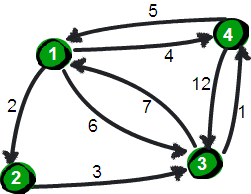上图中有4个城市8条公路，公路上的数字表示这条公路的长短。请注意这些公路是单向的。我们现在需要求任意两个城市之间的最短路程，也就是求任意两个点之间的最短路径。这个问题这也被称为“多源最短路径”问题。
现在需要一个数据结构来存储图的信息，我们仍然可以用一个4*4的矩阵（二维数组e）来存储。比如1号城市到2号城市的路程为2，则设e的值为2。2号城市无法到达4号城市，则设置e的值为∞。另外此处约定一个城市自己是到自己的也是0，例如e为0，具体如下。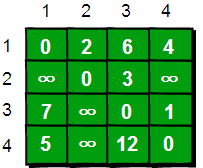现在回到问题：如何求任意两点之间最短路径呢？通过之前的学习我们知道通过深度或广度优先搜索可以求出两点之间的最短路径。所以进行n2遍深度或广度优先搜索，即对每两个点都进行一次深度或广度优先搜索，便可以求得任意两点之间的最短路径。可是还有没有别的方法呢？
我们来想一想，根据我们以往的经验，如果要让任意两点（例如从顶点a点到顶点b）之间的路程变短，只能引入第三个点（顶点k），并通过这个顶点k中转即a->k->b，才可能缩短原来从顶点a点到顶点b的路程。那么这个中转的顶点k是1~n中的哪个点呢？甚至有时候不只通过一个点，而是经过两个点或者更多点中转会更短，即a->k1->k2b->或者a->k1->k2…->k->i…->b。比如上图中从4号城市到3号城市（4->3）的路程e原本是12。如果只通过1号城市中转（4->1->3），路程将缩短为11（e+e=5+6=11）。其实1号城市到3号城市也可以通过2号城市中转，使得1号到3号城市的路程缩短为5（e+e=2+3=5）。所以如果同时经过1号和2号两个城市中转的话，从4号城市到3号城市的路程会进一步缩短为10。通过这个的例子，我们发现每个顶点都有可能使得另外两个顶点之间的路程变短。好，下面我们将这个问题一般化。
当任意两点之间不允许经过第三个点时，这些城市之间最短路程就是初始路程，如下。如现在只允许经过1号顶点，求任意两点之间的最短路程，应该如何求呢？只需判断e[i]+e[j]是否比e[i][j]要小即可。e[i][j]表示的是从i号顶点到j号顶点之间的路程。e[i]+e[j]表示的是从i号顶点先到1号顶点，再从1号顶点到j号顶点的路程之和。其中i是1n循环，j也是1n循环，代码实现如下。
for(i=1;i e[i]+e[j] )
e[i][j] = e[i]+e[j];
}
}

在只允许经过1号顶点的情况下，任意两点之间的最短路程更新为：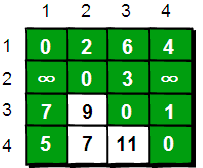通过上图我们发现：在只通过1号顶点中转的情况下，3号顶点到2号顶点（e）、4号顶点到2号顶点（e）以及4号顶点到3号顶点（e）的路程都变短了。
接下来继续求在只允许经过1和2号两个顶点的情况下任意两点之间的最短路程。如何做呢？我们需要在只允许经过1号顶点时任意两点的最短路程的结果下，再判断如果经过2号顶点是否可以使得i号顶点到j号顶点之间的路程变得更短。即判断e[i]+e[j]是否比e[i][j]要小，代码实现为如下。
//经过1号顶点
for(i=1;i e[i]+e[j])  e[i][j]=e[i]+e[j];
//经过2号顶点
for(i=1;i e[i]+e[j])  e[i][j]=e[i]+e[j];

在只允许经过1和2号顶点的情况下，任意两点之间的最短路程更新为：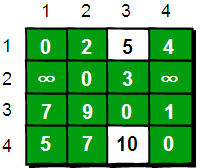通过上图得知，在相比只允许通过1号顶点进行中转的情况下，这里允许通过1和2号顶点进行中转，使得e和e的路程变得更短了。
同理，继续在只允许经过1、2和3号顶点进行中转的情况下，求任意两点之间的最短路程。任意两点之间的最短路程更新为：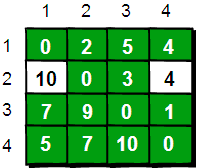最后允许通过所有顶点作为中转，任意两点之间最终的最短路程为：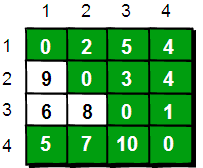整个算法过程虽然说起来很麻烦，但是代码实现却非常简单，核心代码只有五行：
for(k=1;k<=n;k++)
for(i=1;i<=n;i++)
for(j=1;j<=n;j++)
if(e[i][j]>e[i][k]+e[k][j])
e[i][j]=e[i][k]+e[k][j];

这段代码的基本思想就是：最开始只允许经过1号顶点进行中转，接下来只允许经过1和2号顶点进行中转……允许经过1~n号所有顶点进行中转，求任意两点之间的最短路程。用一句话概括就是：从i号顶点到j号顶点只经过前k号点的最短路程。其实这是一种“动态规划”的思想，关于这个思想我们将在《啊哈！算法2——伟大思维闪耀时》在做详细的讨论。下面给出这个算法的完整代码：
#include <stdio.h>
int main()
{
int e,k,i,j,n,m,t1,t2,t3;
int inf=99999999; //用inf(infinity的缩写)存储一个我们认为的正无穷值
//读入n和m，n表示顶点个数，m表示边的条数
scanf("%d %d",&n,&m);

//初始化
for(i=1;i<=n;i++)
for(j=1;j<=n;j++)
if(i==j) e[i][j]=0;
else e[i][j]=inf;
//读入边
for(i=1;i<=m;i++)
{
scanf("%d %d %d",&t1,&t2,&t3);
e[t1][t2]=t3;
}

//Floyd-Warshall算法核心语句
for(k=1;k<=n;k++)
for(i=1;i<=n;i++)
for(j=1;j<=n;j++)
if(e[i][j]>e[i][k]+e[k][j] )
e[i][j]=e[i][k]+e[k][j];

//输出最终的结果
for(i=1;i<=n;i++)
{
for(j=1;j<=n;j++)
{
printf("%10d",e[i][j]);
}
printf("\n");
}

return 0;
}

有一点需要注意的是：如何表示正无穷。我们通常将正无穷定义为99999999，因为这样即使两个正无穷相加，其和仍然不超过int类型的范围（C语言int类型可以存储的最大正整数是2147483647）。在实际应用中最好估计一下最短路径的上限，只需要设置比它大一点既可以。例如有100条边，每条边不超过100的话，只需将正无穷设置为10001即可。如果你认为正无穷和其它值相加得到一个大于正无穷的数是不被允许的话，我们只需在比较的时候加两个判断条件就可以了，请注意下面代码中带有下划线的语句。
//Floyd-Warshall算法核心语句
for(k=1;k<=n;k++)
for(i=1;i<=n;i++)
for(j=1;j<=n;j++)
if(e[i][k]<inf && e[k][j]<inf && e[i][j]>e[i][k]+e[k][j])
e[i][j]=e[i][k]+e[k][j];

上面代码的输入数据样式为：
4 8
1 2 2
1 3 6
1 4 4
2 3 3
3 1 7
3 4 1
4 1 5
4 3 12

第一行两个数为n和m，n表示顶点个数，m表示边的条数。
接下来m行，每一行有三个数t1、t2 和t3，表示顶点t1到顶点t2的路程是t3。
得到最终结果如下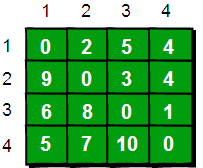通过这种方法我们可以求出任意两个点之间最短路径。它的时间复杂度是O(N3)。令人很震撼的是它竟然只有五行代码，实现起来非常容易。正是因为它实现起来非常容易，如果时间复杂度要求不高，使用Floyd-Warshall来求指定两点之间的最短路或者指定一个点到其余各个顶点的最短路径也是可行的。当然也有更快的算法，请看下一节：Dijkstra算法。
另外需要注意的是：Floyd-Warshall算法不能解决带有“负权回路”（或者叫“负权环”）的图，因为带有“负权回路”的图没有最短路。例如下面这个图就不存在1号顶点到3号顶点的最短路径。因为1->2->3->1->2->3->…->1->2->3这样路径中，每绕一次1->-2>3这样的环，最短路就会减少1，永远找不到最短路。其实如果一个图中带有“负权回路”那么这个图则没有最短路。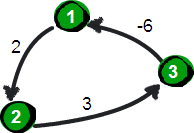展开全文数据结构 最短路径 Dijkstra算法 Floyd算法
• ## 图的五种最短路径算法

万次阅读 多人点赞 2018-04-14 16:46:38
本文总结了图的几种最短路径算法的实现：深度或广度优先搜索算法，费罗伊德算法，迪杰斯特拉算法，Bellman-Ford 算法。1）深度或广度优先搜索算法（解决单源最短路径）从起点开始访问所有深度遍历路径或广度优先路径...
本文总结了图的几种最短路径算法的实现：深度或广度优先搜索算法，费罗伊德算法，迪杰斯特拉算法，Bellman-Ford 算法。1）深度或广度优先搜索算法（解决单源最短路径）从起点开始访问所有深度遍历路径或广度优先路径，则到达终点节点的路径有多条，取其中路径权值最短的一条则为最短路径。下面是核心代码：void dfs(int cur,int dst){
if(minpath<dst) return;//当前走过的路径大雨之前的最短路径，没有必要再走下去了
if(cur==en){//临界条件，当走到终点n
if(minpath>dst){
minpath=dst;
return;
}
}
for(int i=1;i<=n;i++){
if(mark[i]==0&&edge[cur][i]!=inf&&edge[cur][i]!=0){
mark[i]=1;
dfs(i,dst+edge[cur][i]);
mark[i]=0;//需要在深度遍历返回时将访问标志置0
}
}
return;
}例：先输入n个节点，m条边，之后输入有向图的m条边，边的前两个元素表示起点和终点，第三个值表示权值，输出1号城市到n号城市的最短距离。#include<bits/stdc++.h>
using namespace std;
#define nmax 110
#define inf 999999999
int minpath,n,m,en,edge[nmax][nmax],mark[nmax];//最短路径，节点数，边数，终点，邻接矩阵，节点访问标记
void dfs(int cur,int dst){
if(minpath<dst) return;//当前走过的路径大雨之前的最短路径，没有必要再走下去了
if(cur==en){//临界条件，当走到终点n
if(minpath>dst){
minpath=dst;
return;
}
}
for(int i=1;i<=n;i++){
if(mark[i]==0&&edge[cur][i]!=inf&&edge[cur][i]!=0){
mark[i]=1;
dfs(i,dst+edge[cur][i]);
mark[i]=0;//需要在深度遍历返回时将访问标志置0
}
}
return;
}
int main ()
{
while(cin>>n>>m&&n!=0){
//初始化邻接矩阵
for(int i=1;i<=n;i++){
for(int j=1;j<=n;j++){
edge[i][j]=inf;
}
edge[i][i]=0;
}
int a,b;
while(m--){
cin>>a>>b;
cin>>edge[a][b];
}
minpath=inf;
memset(mark,0,sizeof(mark));
mark=1;
en=n;
dfs(1,0);
cout<<minpath<<endl;
}
}
程序运行结果如下：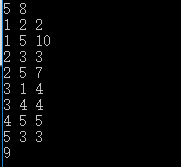2）弗洛伊德算法（解决多源最短路径）：时间复杂度o(n^3),空间复杂度o(n^2)基本思想：最开始只允许经过1号顶点进行中转，接下来只允许经过1号和2号顶点进行中转.......允许经过1~n号所有顶点进行中转，来不断动态更新任意两点之间的最短距离。即求从i号顶点到j顶点只经过前k号点的最短距离。分析如下：1，首先构建邻接矩阵edge[n+1][n+1],假如现在只允许经过1号节点，求任意两点间的最短距离，很显然edge[i][j]=min(edge[i][j],edge[i]+edge[j]),代码如下：for(int i=1;i<=n;i++){
for(int j=1;j<=n;j++){
if(edge[i][j]>edge[i]+edge[j]){
edge[i][j]=edge[i]+edge[j];
}
}
}2.接下来继续求在只允许经过1和2号两个顶点的情况下任意两点之间的最短距离，在已经实现了从i号顶点到j号顶点只经过前1号点的最短路程的前提下，现在插入第2号节点，来看看能不能更新最短路径，因此只需在步骤一求得的基础上，进行edge[i][j]=min(edge[i][j],edge[i]+edge[j]);.......3.很显然，需要n次这样的更新，表示依次插入了1号2号.......n号节点，最后求得的edge[i][j]是从i号顶点到j号顶点只经过前n号点的最短路程。因此核心代码如下：#include<bits/stdc++.h>
using namespace std;
#define inf 999999999
int main ()
{
for(int k=1;k<=n;k++){
for(int i=1;i<=n;i++){
for(int j=1;j<=n;j++){
if(edge[k][j]<inf&&edge[i][k]<inf&&edge[i][j]>edge[i][k]+edge[k][j]){
edge[i][j]=edge[i][k]+edge[k][j];
}
}
}
}
}
例1：寻找最短的从商场到赛场的路线。其中商店在1号节点处，赛场在n号节点处，1~n节点中有m条线路双向连接。/***先输入n，m，在输入m个三元组，n为路口数，m表示有几条路，其中1为商店，n为赛场，三元组分别表示起点终点，和该路径长，输出1到n的最短距离***/#include<bits/stdc++.h>
using namespace std;
#define inf 999999999
#define nmax 110
int n,m,edge[nmax][nmax];
int main ()
{
int a,b;
while(cin>>n>>m&&n!=0){
for(int i=1;i<=n;i++){
for(int j=1;j<=n;j++){
edge[i][j]=inf;
}
edge[i][i]=0;
}
while(m--){
cin>>a>>b;
cin>>edge[a][b];
edge[b][a]=edge[a][b];
}
for(int k=1;k<=n;k++){
for(int i=1;i<=n;i++){
for(int j=1;j<=n;j++){
if(edge[k][j]<inf&&edge[i][k]<inf&&edge[i][j]>edge[i][k]+edge[k][j]){
edge[i][j]=edge[i][k]+edge[k][j];
}
}
}
}
cout<<edge[n]<<endl;
}
}
程序运行结果如下：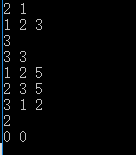3）迪杰斯特拉算法（解决单源最短路径）基本思想：每次找到离源点（如1号节点）最近的一个顶点，然后以该顶点为中心进行扩展，最终得到源点到其余所有点的最短路径。基本步骤：1，设置标记数组book[]：将所有的顶点分为两部分，已知最短路径的顶点集合P和未知最短路径的顶点集合Q，很显然最开始集合P只有源点一个顶点。book[i]为1表示在集合P中；2，设置最短路径数组dst[]并不断更新：初始状态下，dst[i]=edge[s][i](s为源点，edge为邻接矩阵),很显然此时dst[s]=0,book[s]=1.此时，在集合Q中可选择一个离源点s最近的顶点u加入到P中。并依据以u为新的中心点，对每一条边进行松弛操作（松弛是指由顶点s-->j的途中可以经过点u，并令dst[j]=min(dst[j],dst[u]+edge[u][j])）,并令book[u]=1;3，在集合Q中再次选择一个离源点s最近的顶点v加入到P中。并依据v为新的中心点，对每一条边进行松弛操作（即dst[j]=min(dst[j],dst[v]+edge[v][j])）,并令book[v]=1;4,重复3，直至集合Q为空。核心代码如下：#include<bits/stdc++.h>
using namespace std;
#define nmax 110
#define inf 999999999
/***构建所有点最短路径数组dst[],且1为源点***/
int u;/***离源点最近的点***/
int minx;
for(int i=1;i<=n;i++) dst[i]=edge[i];
for(int i=1;i<=n;i++) book[i]=0;
book=1;
for(int i=1;i<=n-1;i++){
minx=inf;
for(int j=1;j<=n;j++){
if(book[j]==0&&dst[j]<minx){
minx=dst[j];
u=j;
}
}
book[u]=1;
/***更新最短路径数组***/
for(int k=1;k<=n;k++){
if(book[k]==0&&dst[k]>dst[u]+edge[u][k]&&edge[u][k]<inf){
dst[k]=dst[u]+edge[u][k];
}
}
}
例1：给你n个点，m条无向边，每条边都有长度d和花费p，给你起点s，终点t，要求输出起点到终点的最短距离及其花费，如果最短距离是有多条路线，则输出花费最少的。输入：输入n，m，点的编号是1~n，然后是m行，每行4个数a，b，d，p，表示a和b之间有一条边，且长度为d，花费为p。最后一行是两个数s，t，起点s，终点t。n和m为0时输入结束。（1<n<=1000,0<m<100000,s!=t）输出：输出一行，有两个数，最短距离及其花费。分析：由于每条边有长度d和花费p，最好构建变结构体存放。使用邻接矩阵求解：#include<bits/stdc++.h>
using namespace std;
#define nmax 110
#define inf 999999999
struct Edge{
int len;
int cost;
}edge[nmax][nmax];
int u,n,m,book[nmax],s,t,dst[nmax],spend[nmax];
int minx;
int main (){
while(cin>>n>>m&&n!=0&&m!=0){
for(int i=1;i<=n;i++){
for(int j=1;j<=n;j++){
edge[i][j].len=inf;
edge[i][j].cost=0;
}
edge[i][i].len=0;
}
int a,b;
while(m--){
cin>>a>>b;
cin>>edge[a][b].len>>edge[a][b].cost;
edge[b][a].len=edge[a][b].len;
edge[b][a].cost=edge[a][b].cost;
}
cin>>s>>t;
for(int i=1;i<=n;i++) {dst[i]=edge[s][i].len;spend[i]=edge[s][i].cost;}
for(int i=1;i<=n;i++) book[i]=0;
book[s]=1;
for(int i=1;i<=n-1;i++){
minx=inf;
for(int j=1;j<=n;j++){
if(book[j]==0&&dst[j]<minx){
minx=dst[j];
u=j;
}
}
book[u]=1;
for(int k=1;k<=n;k++){
if(book[k]==0&&(dst[k]>dst[u]+edge[u][k].len||(dst[k]==dst[u]+edge[u][k].len&&spend[k]>spend[u]+edge[u][k].cost))&&edge[u][k].len<inf){
dst[k]=dst[u]+edge[u][k].len;
spend[k]=spend[u]+edge[u][k].cost;
}
}
}
cout<<dst[t]<<' '<<spend[t]<<endl;
}
}程序运行结果如下：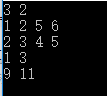4）Bellman-Ford算法（解决负权边，解决单源最短路径，前几种方法不能解决负权边）主要思想：所有的边进行n-1轮松弛，因为在一个含有n个顶点的图中，任意两点之间的最短路径最多包含n-1条边。换句话说，第1轮在对所有的边进行松弛操作后，得到从1号顶点只能经过一条边到达其余各定点的最短路径长度，第2轮在对所有的边进行松弛操作后，得到从1号顶点只能经过两条边到达其余各定点的最短路径长度，........此外，Bellman-Ford算法可以检测一个图是否含有负权回路：如果经过n-1轮松弛后任然存在dst[e[i]]>dst[s[i]]+w[i].例1：对图中含有负权的有向图，输出从1号节点到各节点的最短距离，并判断有无负权回路。#include<bits/stdc++.h>
using namespace std;
#define nmax 1001
#define inf 999999999
int n,m,s[nmax],e[nmax],w[nmax],dst[nmax];
int main ()
{
while(cin>>n>>m&&n!=0&&m!=0){
for(int i=1;i<=m;i++){
cin>>s[i]>>e[i]>>w[i];
}
for(int i=1;i<=n;i++)
dst[i]=inf;
dst=0;
for(int i=1;i<=n-1;i++){
for(int j=1;j<=m;j++){
if(dst[e[j]]>dst[s[j]]+w[j]){
dst[e[j]]=dst[s[j]]+w[j];
}
}
}
int flag=0;
for(int i=1;i<=m;i++){
if(dst[e[i]]>dst[s[i]]+w[i]){
flag=1;
}
}
if(flag) cout<<"此图有负权回路"<<endl;
else{
for(int i=1;i<=n;i++){
if(i==1) cout<<dst[i];
else cout<<' '<<dst[i];
}
cout<<endl;
}
}
}
程序运行结果如下：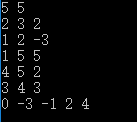5）SPFA(Shortest Path Faster Algorithm)算法是求单源最短路径的一种算法，它是Bellman-ford队列优化，它是一种十分高效的最短路算法。实现方法：建立一个队列，初始时队列里只有起始点s，在建立一个数组记录起始点s到所有点的最短路径（初始值都要赋为极大值，该点到他本身的路径赋为0）。然后执行松弛操作，用队列里的点去刷新起始点s到所有点的距离的距离，如果刷新成功且刷新的点不在队列中，则把该点加入到队列，重复执行直到队列为空。此外，SPFA算法可以判断图中是否有负权欢=环，即一个点的入队次数超过N。#include<bits/stdc++.h>
using namespace std;
int n,m,len;
struct egde{
int to,val,next;
}e;
e[len].to=to;
e[len].val=val;
len++;
}
void spfa()
{
queue<int>q;
q.push(1);
vis=1;
while(!q.empty())
{
int t=q.front();
q.pop();
vis[t]=0;
int s=e[i].to;
if(dis[s]>dis[t]+e[i].val){
dis[s]=dis[t]+e[i].val;
if(vis[s]==0){
q.push(s);
vis[s]=1;
}
}
}
}

}
int main(){
int from,to,val;
while(cin>>n>>m){
memset(vis,0,sizeof(vis));
/* for(int i=1;i<=n;i++){
dis[i]=99999999;
}*/
memset(dis,0x3f,sizeof(dis));
dis=0;len=1;
for(int i=0;i<m;i++){
cin>>from>>to>>val;
}
spfa();
for(int i=1;i<=n;i++){
cout<<dis[i]<<" ";
}
cout<<endl;
}
}

展开全文• 1 最短路径概念1.1 定义官方定义：对于内网图而言，最短路径是指两顶点之间经过的边上权值之和最小的路径。并且我们称路径上的第一个顶点为源点，最后一个顶点为终点。由于非内网图没有边上的权值，所谓的最短路径...数据结构
• 迪杰斯特拉(Dijkstra)算法是典型最短路径算法，用于计算一个节点到其他节点的最短路径。 它的主要特点是以起始点为中心向外层层扩展(广度优先搜索思想)，直到扩展到终点为止。算法思想 每次找到离源点最近的一个...dijkstra
• 问题：根据每条边的权值，求出从起点s到其他每个顶点的最短路径最短路径的长度。 说明：不考虑权值为负的情况，否则会出现负值圈问题。 s：起点 v：算法当前分析处理的顶点 w：与v邻接的顶点 dvdvd_v：从s到v...
• ## 最短路径四大算法

万次阅读 多人点赞 2017-11-08 20:13:53
熟悉的最短路算法就几种：bellman-ford，dijkstra，spfa，floyd。首先说明一点，就是关于负环的问题。 bellman-ford可以用于边权为负的图中，图里有负环也可以，如果有负环，算法会检测出负环。 时间复杂度O（VE）...最短路径算法
• ## 几个最短路径的算法

万次阅读 多人点赞 2018-06-08 22:12:34
思想： Floyd算法的基本思想如下：从任意节点A到任意节点B的最短路径不外乎2种可能，1是直接从A到B，2是从A经过若干个节点X到B。所以，我们假设Dis(AB)为节点A到节点B的最短路径的距离，对于每一个节点X，我们检查...
• ## 图的四种最短路径算法

万次阅读 多人点赞 2019-07-05 10:03:49
本文总结了图的几种最短路径算法的实现：深度或广度优先搜索算法，弗洛伊德算法，迪杰斯特拉算法，Bellman-Ford算法1），深度或广度优先搜索算法（解决单源最短路径）从起始结点开始访问所有的深度遍历路径或广度...
• 本文总结了图的几种最短路径算法的实现：深度或广度优先搜索算法，弗洛伊德算法，迪杰斯特拉算法，Bellman-Ford算法 1），深度或广度优先搜索算法（解决单源最短路径） 从起始结点开始访问所有的深度遍历路径...迪杰斯特拉 弗洛伊德算法 图的最短路径 算法
• 有向图最短路径问题---Dijkstra算法（过程）有向图 最短路径 Dijkstra算法
• 基本思想：Dijkstra算法（迪杰斯特拉算法）是解单源最短路径问题的贪心算法。 Dijkstra算法特点：以起始点为中心向外层层扩展，直到扩展到终点为止，是一种广度优先搜索方法。 Dijkstra算法原理：最优子路径存...algorithm
• 必经点最短路径问题求解，遗传算法及MIP求解模型遗传算法 MIP
• Dijkstra：O(n2)适用于权值为非负的图的单源最短路径，用斐波那契堆的复杂度O(E+VlgV)， BellmanFord：适用于权值有负值的图的单源最短路径，并且能够检测负圈，复杂度O(VE) SPFA：适用于权值有负值，且没有负圈的...dijkstra 数据结构
• 最短路径问题总结 图中还有些地方没有完善，但是一时也没没办法解决，希望大家知道的能够提供一下表中不足的地方，万分感谢！...最短路径算法（三）–[ 带负权值图 ]Bellman-Flod的解法（JAVA ）最短路径 JAVA
• ## 动态规划算法--最短路径问题

万次阅读 多人点赞 2016-11-16 09:27:53
将用Dijkstra算法解决最短路径问题。 最短路径有一个重要特性： 如果由起点A经过P点和H点而到达终点G是一条最短路线，则由点P出发经过H点到达终点G的这条子路线，对于从点 P出发到达终点的所有可能选择的不同路线来...动态规划 dijkstra 最短路径
• 首先介绍这三个概念，很多人都听过最短路径了，但是最短路径树却很少听过，关于最短路径树的介绍也不太多。而最短路径树和最小生成树更是完全不同的两个概念。 最短路径就是从一个指定的顶点出发，计算从该顶点出发...最短路径 最短路径树 最小生成树
• 最短路径算法主要有二 Dijkstra算法 Floyd算法 Dijkstra算法研究的是从初始点到其他每一结点的最短路径 而Floyd算法研究的是任意两结点之间的最短路径 以下图为例，首先介绍Dijstra的原理 红字为各结点的...DIJKSTRA MATLAB
• 由于从一顶点到另一顶点可能存在着多条路径，每条路径上所经过的边数可能不同，即路径长度不同，我们把路径长度最短（即经过的边数最少）的那条路径叫做最短路径，其路径长度叫做最短路径长度或最短距离。...
...